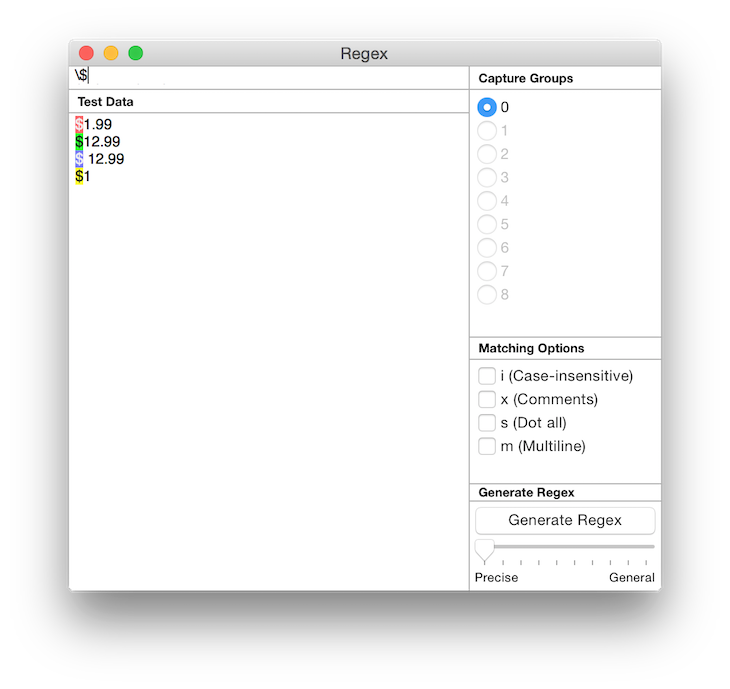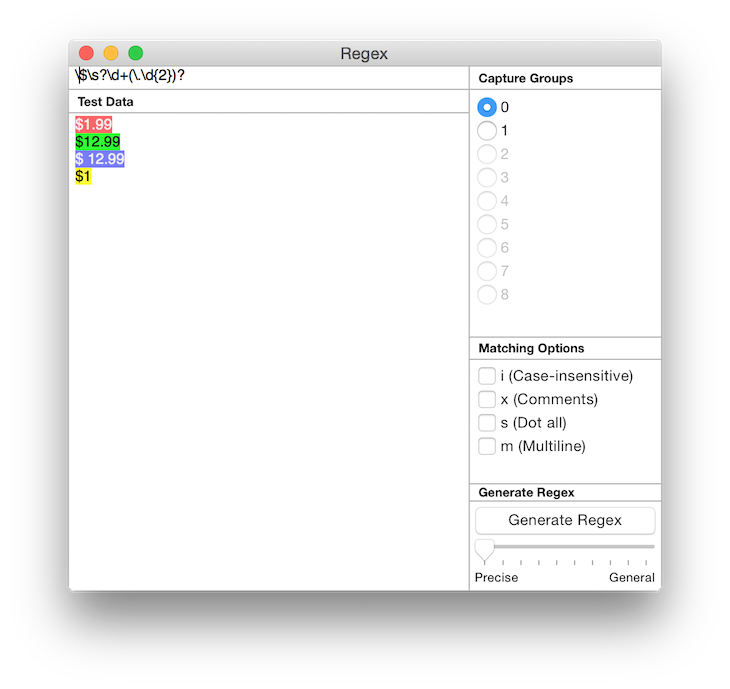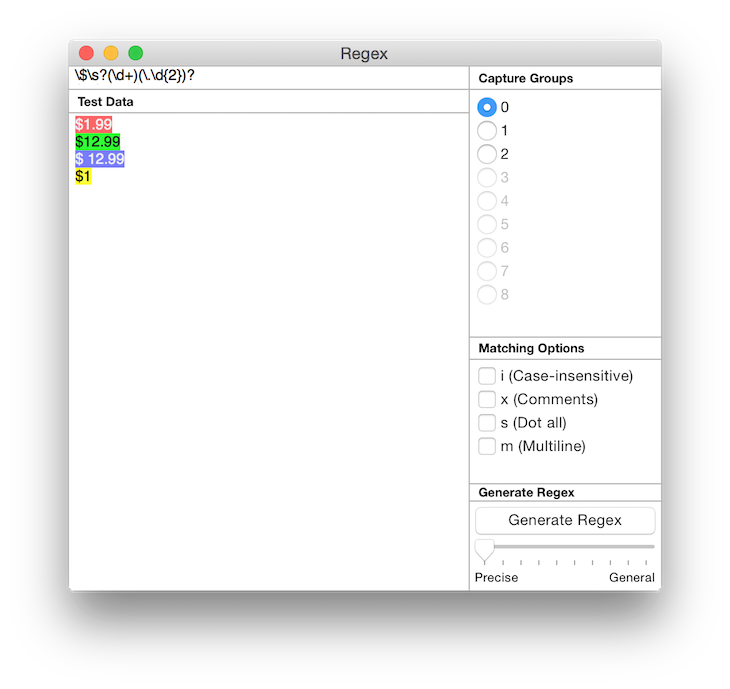Regex for Mac OS X Tutorial

# Regex for Mac OS X Tutorial

Regular expressions or regex (also referred to as regexp) is a way to write a string of text that will serve as a pattern to match another string (or strings). Regex is terse and well supported by many tools including popular programming languages such as Javascript, Java, Perl, Ruby and Python, as well as shell tools such as sed and text editors such as TextMate, vi and Emacs.

There are many ways which regex can be useful, including input validation, extracting strings from text, and search and replacing of text.

## Input Validation

Regex is incredibly handy when you want to perform validation of input from HTML forms. For example, you might want to parse emails with expressions such as

``````.+@.{2,}\..{2,}
``````

Or URLs with

``````https?://(\w+)\.(\w{2,}/?(\S*)?)
``````

## Matching Text

You can match prices such as \$1.99 with

``````\\$\d+(\.\d{1,2})?
``````

## How to build and test a regular expression using Regex for Mac OS X

We'll take matching prices as an example of building a regex and testing it with Regex for Mac OS X (free trial). Here's a few steps to do it.

1. Come up with examples of the string you want to match against, typing them into the sample area. So you would have

``````\$1.99
\$12.99
\$ 12.99
\$1
``````2. Come up with a regex and try to match them to the first example and modify the regex to also work with each example as you work down the list. To match a \$ we need to escape it with a preceding \ so we start with

``````\\$
``````3. \d matches a digit, so let's match \$1 with

``````\\$\d
``````

4. We want to match the . in \$1. remembering that . has to be escaped with a preceding \ too. (Otherwise . matches any character)

``````\\$\d\.
``````

5. Matching \$1.99 we then have. This now matches the first example

``````\\$\d\.\d\d
``````

6. Since we can match a repeating pattern with {}, let us use it here to make it clear that there is exactly 2 ending digits. And we are now done with the first example

``````\\$\d\.\d{2}
``````7. In the 2nd example, we observe that the dollar value can sometimes be more than 1 digit. So let's use the + quantifier to indicate we want 1 or more repeats. This expression matches both examples

``````\\$\d+\.\d{2}
``````

8. For the 3rd example, we notice there can be an optional space between the \$ and first digit. We'll make use of \s as a whitespace matching character as well as the ? quantifier to indicate it is optional.

``````\\$\s?\d+\.\d{2}
``````

9. And finally, we notice that the cents part (.99) is optional too. So let's make it so with

``````\\$\s?\d+(\.\d{2})?
``````And this matches every one of the examples above.

10. Let's extend this example further. Perhaps you want to also match the dollar value in the price. We can do

``````\\$\s?(\d+)(\.\d{2})?
``````Notice that under Capture Group on the right, the radio buttons for 1 and 2 are now available? Press on 1. The dollar value will now be highlighted in the sample text area instead of the entire price. If you press 2, it will highlight the cents value. This is because capture group 0 refers to the string matching the entire expression, and capture groups 1, 2 and onwards refer to the string matching each parenthesis () group counting from the left.## More

There are additional features of regular expressions that can help with otherwise difficult to write expressions.

Positive Lookahead forces a pattern to match only if it is followed by another pattern. i.e. the lookahead pattern is the suffix. Eg, given the source text:

``````aaaaaa111bbb222
``````

This will have 3 matches for "aa", the indices [0,2], [2,4], [4,6].

``````aa
``````

What if you only want to match the "aa" that is followed by "111"? The following regex will match only index [4,6]:

``````aa(?=111)
``````

Note that this is different from the following which will match the index [4,9].

``````aa(111)
``````

Negative Lookahead forces a pattern to match only if it is not followed by another pattern. E.g given the same source text:

``````aaaaaa111bbb222
``````

What if you want to match both the "aa" that is not followed by "111"? The following regex will do it, using negative lookahead:

``````aa(?!111)
``````

## Positive Lookbehind

Positive Lookbehind works like Positive Lookahead, except the lookbehind expression is the prefix instead of the suffix. Given the same source text:

``````aaaaaa111bbb222
``````

The following will match "111" and not "222":

``````(?<=a)\d{3}
``````

## Negative Lookbehind

Similarly, Negative Lookbehinds matches only if the prefix doesn't match.

``````aaaaaa111bbb222
``````

The following will match "222" and not "111":

``````(?<!a)\d{3}
``````

## Backreferences

Backreference is a placeholder for a previous match. This is useful where you have your target text surrounded by delimiting text. E.g. HTML tags (not that you should use Regular expressions for HTML as a matter of habit).

Given this source:

``````Something here <span>this is matched</span>. another thing.
``````

This will match the span tag and contents:

``````<(\w*)>.*</\1>
``````

Notice the `\1` backreference. `\1` references to the first capture group, `\2` for the 2nd and so on.

If you need to use regular expressions in programming languages like Javascript, you will need to escape \ with an additional \ . For e.g. this expression

``````\w{2}
``````

needs to be specified as the following in certain programming languages (including Javascript)

``````\\w{2}
``````

Regex for Mac OS X automatically does this escaping for you when you copy regex from the the app and automatically unescapes (removing double \) when you paste into the app.

## Conclusion

This tutorial gives a simple introduction to building regular expressions using Regex for Mac OS X. You might have noticed that the price regex developed only matches \$. What about pounds and other currencies? And you might find that the URL regex is overly simplistic. After working with regular expressions for some time, you will discover that they are very powerful and also needs to be used carefully. For example, see here for a much more accurate regex for matching URLs. It is very long and complex, making it hard to modify. For a good overview of regex, see the Wikipedia article for Regular Expression.

Interested in exploring regular expressions further? Check out the free trial of Regex for OS X:

Want more articles like this in your inbox? Join our newsletter to receive free email updates, tips and tricks related to iOS & OS X.